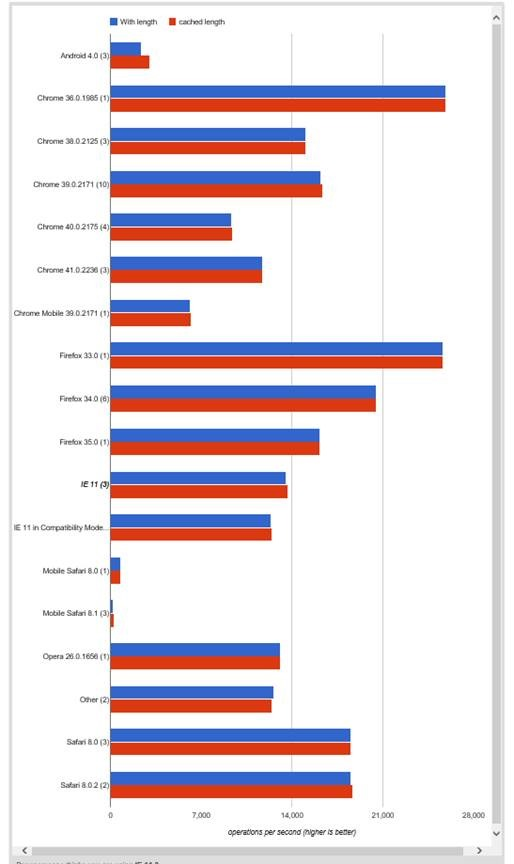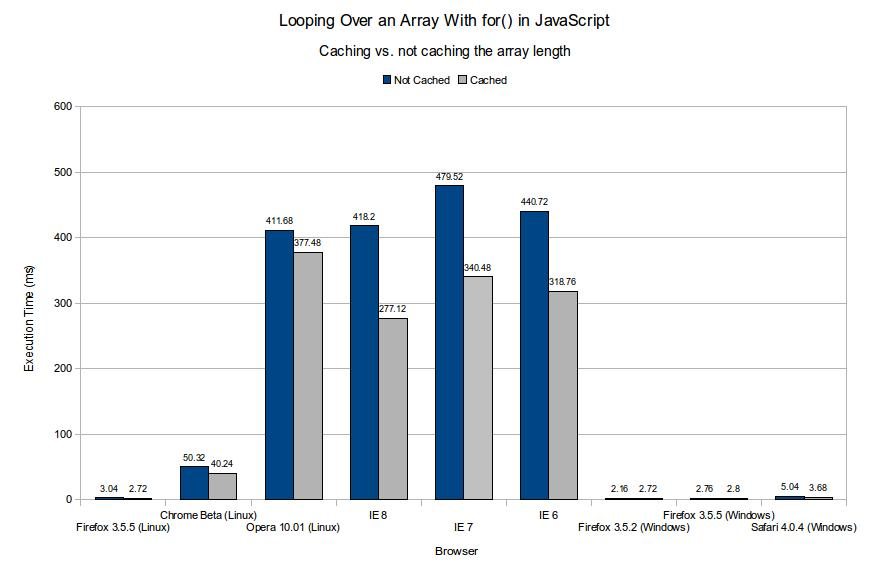# JavaScript 有必要缓存 for 循环中的 Array.length 吗？

"TL;DR: No"

## 问题

``````// 不缓存
for (var i = 0; i < arr.length; i++) {
...
}

// 缓存
var len = arr.length;
for (var i = 0; i < len; i++) {
...
}``````

## 理由

### 从测试结果上看

stackoverflow 上也有这个讨论，For-loop performance: storing array length in a variable

accepted 的答案是说缓存会起到加速的结果，给出了 jsPerf 测试。### 从 V8 的中间代码分析

``````function uncached(arr) {
for (var i = 0; i < arr.length; i++) {
arr[i]
}
}``````

``````function BLACKHOLE(sum, arr) {
try { } catch (e) { }
}

function uncached(arr) {
var sum = 0;
for (var i = 0; i < arr.length; i++) {
sum += arr[i];
if (sum < 0) BLACKHOLE(arr, sum);
}
return sum;
}``````

【完】